Definitions of Square Dance Calls and Concepts
"Z" Concept [C3B]

Index -->  Plus  |  A1  |  A2  |  C1  |  C2  |  C3A  |  C3B  |  C4  |  NOL  |
Definitions (Text Only) -->  Plus  |  A1  |  A2  |  C1  |  C2  |  C3A  |  C3B  |  C4  |  NOL  |
 Find call:

 \$B8@8l(BA "Z" is a 2 x 2 that is offset by one position (e.g., the formation obtained from a Mini-Wave Box after 1/2 Press Ahead). Work in the Offset 2 x 2 and maintain the footprints. EN: 10
"Z" \$B\$O(B 2 x 2 \$B\$,(B 1 \$B%]%8%7%g%s(B offset \$B\$7\$?(B formation \$B\$G\$9(B (\$BNc\$(\$P(B, Mini Wave \$B\$N(B Box \$B\$+\$i(B 1/2 Press Ahead \$B\$r\$7\$FF@\$i\$l\$k(B formation). Offset 2 x 2 \$B\$GF0\$-(B, 4 \$B?M\$N%@%s%5!<\$N0LCV\$rJ]\$A\$^\$9(B. JP: 10

"Z" Walk & Dodge: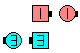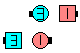"Z" Walk & Dodge\$B\$NA0(B \$B8e(B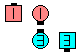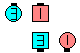"Z" Walk & Dodge\$B\$NA0(B \$B8e(B

"Z" Counter Rotate 1/4: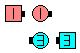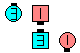"Z" Counter Rotate 1/4\$B\$NA0(B \$B8e(B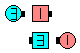"Z" Counter Rotate 1/4\$B\$NA0(B \$B8e(B

\$BCm

• All "Z" calls must end on footprints. Shape-changing calls are not allowed. EN: 20
\$BA4\$F\$N(B "Z" \$B%3!<%k\$O(B, \$B;O\$a\$N(B 4 \$B?M\$N0LCV\$G=*\$o\$i\$M\$P\$J\$j\$^\$;\$s(B. Shape-change \$B\$N%3!<%k\$O5v\$5\$l\$^\$;\$s(B. JP: 20
• Be careful; sometimes seeing a "Z" is very difficult. There is always someone directly side-by-side someone else, or directly in front of or behind someone else. This is the 'bending' point of your "Z". Reach forward (to the side) or behind (to the side) if necessary to touch your partner in the "Z", but do not physically move from your spot. Maintaining precise positioning is crucial! EN: 30
\$B\$H\$-\$I\$-(B, "Z" \$B\$,\$H\$F\$bFq\$7\$/\$J\$C\$F\$k\$3\$H\$r8+\$+\$1\$^\$9\$N\$G(B, \$BCm0U\$7\$F\$/\$@\$5\$\$(B. \$B??2#\$^\$?\$O(B, \$B??\$sA0\$+??8e\$m\$KJB\$s\$G\$\$\$k?M\$,I,\$:\$\$\$^\$9(B. \$B\$=\$3\$,(B "Z" \$B\$N6J\$,\$C\$F\$\$\$k\$H\$3\$m\$G\$9(B. \$B2#\$KJB\$s\$G\$\$\$k\$H\$-(B, \$BI,MW\$J\$i(B, \$Bl=j\$+\$iF0\$\$\$F\$O\$\$\$1\$^\$;\$s(B. \$B@53N\$J0LCV\$rJ];}\$9\$k\$3\$H\$,\$H\$F\$b=EMW\$G\$9(B. JP: 30
• \$BDiagonal Box [C3B],

which is a 2 x 2 that is offset by two positions. EN: 40
2 x 2 \$B\$,(B 2 \$B%]%8%7%g%s(B offset \$B\$7\$?(B formation \$B\$G\$9(B. JP: 40Choreography for "Z" ConceptComments? Questions? Suggestions?